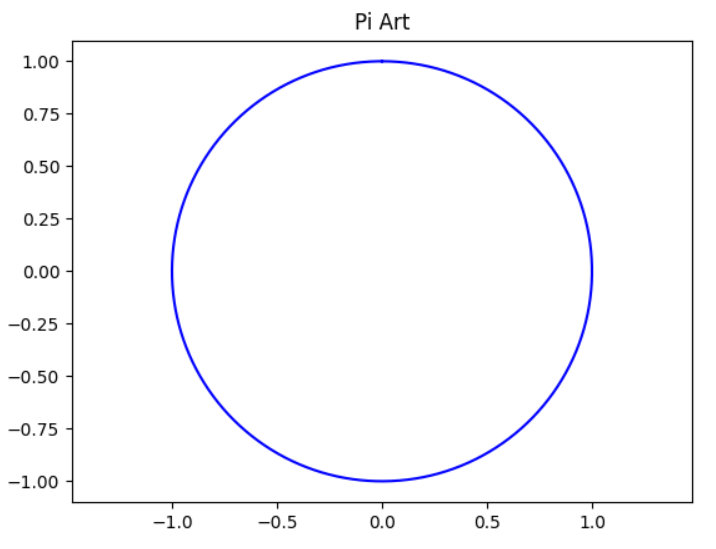Tutorial

## How to use Pi Constant in Python?

##### Python Tutor

In the realm of programming and mathematics, few constants hold as much significance as the mathematical wonder known as π (pronounced as “pi”). Its influence extends across a multitude of disciplines.

When it comes to harnessing pi in the world of Python programming, a treasure trove of possibilities unfolds.

In this article, we will learn Pi in Python, and reveal its various applications and the sheer magic it can bring to our Python code with examples.

## What is Pi?

### Defining Pi or π

Pi (π) is an irrational number that signifies the ratio of a circle’s circumference to its diameter. Approximately equal to 3.14159265358979323846… (and the digits go on forever without repeating).

Pi is one of the most famous and intriguing constants in the world of mathematics. It is essential for a wide array of mathematical calculations and has been studied and approximated for thousands of years.

### The Universal Nature of Pi

Pi is not confined to the realm of mathematics alone.

Its omnipresence stretches far and wide. From geometry to physics, engineering to architecture, and even music to art, π leaves its indelible mark on the fabric of our world.

In Python, its applications are equally diverse, making it a fundamental tool in a programmer’s arsenal.

## Calculating Pi in Python

### Pi Approximation with Python

Python offers various methods to approximate Pi or π.

Let’s delve into a few approaches:

#### 1. Using the `math` Library

Python Pi can be accessed conveniently using the `math` library.

By simply importing it and referencing `math.pi`, you can retrieve the precise value of Pi(π) in Python.

Here’s how you can use it:

``````import math

python_pi = math.pi
print(python_pi)``````

This simple code snippet will output the value of Pi (π), demonstrating the elegance of math.pi in Python.

#### 2. The Monte Carlo Method

For those seeking a more adventurous approach to obtaining Python pi, the Monte Carlo method is an intriguing way to approximate π.

It leverages randomness and probability, making it a unique way to get pi in Python.

Consider this Python code:

``````import random

def monte_carlo_pi(num_samples):
inside_circle = 0

for _ in range(num_samples):
x = random.random()
y = random.random()

if x**2 + y**2 <= 1:
inside_circle += 1

return 4 * inside_circle / num_samples

pi_approximation = monte_carlo_pi(1000000)
print(pi_approximation) #3.142624
``````

This code utilizes random numbers to estimate Python pi, showcasing Python’s versatility in handling complex mathematical tasks.

### Pi Precision Matters

Depending on your project’s requirements, you may need varying levels of precision when approximating Pi. Python, with its rich ecosystem of libraries and custom functions, allows you to fine-tune your calculations to meet your specific needs.

## Pi’s Role in Scientific Computing

### Scientific Python Libraries

Python is the go-to language for scientific and numerical computing and Pi plays a pivotal role in these domains.

Various Python libraries, such as NumPy and SciPy, leverage Pi extensively in their computations.

Whether you are dealing with complex mathematical models, data analysis, or simulations, Pi is always at your disposal.

## Art and Pi: A Creative Connection

### Generating Visual Art in Python using Pi

Python’s capabilities extend beyond calculations and data processing; it can be a powerful tool for creating visual art. When combined with the beauty of Pi, the results can be nothing short of mesmerizing.

Let’s explore the connection between art and Pi in Python.

#### Fractal Art

Fractals, intricate geometric patterns, can be generated with Python using mathematical algorithms, many of which involve how to use pi in Python.

These algorithms often rely on math.pi in Python as a key component.

By harnessing the principles of chaos theory and self-similarity, you can create stunning fractal art.

The famous Mandelbrot set is a prime example.

#### Pi Art with matplotlib

The `matplotlib` library in Python empowers you to create intricate plots and visualizations with ease.

Here’s a simple example of generating Pi Art using `matplotlib`:

``````import matplotlib.pyplot as plt
import numpy as np

# Define the angles from 0 to 2π
theta = np.linspace(0, 2 * np.pi, 1000)

# Create a mesmerizing Pi Art
x = np.sin(theta)
y = np.cos(theta)

plt.plot(x, y, color='blue')
plt.title('Pi Art')
plt.axis('equal')
plt.show()
``````

This code snippet showcases the beauty that can be created with Pi as your artistic muse, exemplifying how to write Piin Python as a work of art.

## Musical Harmony and Pi

### The Fibonacci Sequence

The Fibonacci sequence, which can be closely related to Pi, has a profound influence on music and aesthetics. This sequence is used to create harmonious compositions and arrangements in music, demonstrating how to use pi in Python for creative expression.

Composers often use the ratios derived from the Fibonacci sequence to create pleasing melodies and rhythms.

## Conclusion

In the realm of programming, mathematics, art, and music, the influence of Pi is undeniable.

Python, with its libraries, allows us to harness the enchanting power of this irrational number in countless ways.

From precise mathematical calculations to stunning visual art and harmonious music, Pi in Python is an endless source of inspiration and creativity.

As you continue your journey in the world of programming, remember that the magic of Pi is always at your fingertips, waiting to be unleashed.

Want to become a Python expert, you might want to learn with an online Python tutor.